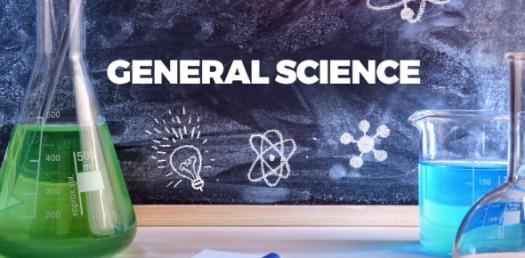# General Science Trivia Quiz Questions

35 Questions | Total Attempts: 975SettingsAre you looking for a general science trivia quiz questions to test out just how much knowledge you have? The quiz below is exactly what you need to refresh your memory and show you which topics you did not understand fully. All the best, and be sure to check out other quizzes like it!

• 1.
The metric system of measurement is based on the which number?
• A.

13

• B.

10

• C.

9

• D.

7

• 2.
The basic unit of length in the metric system is the
• A.

Meter

• B.

Centimeter

• C.

Milimeter

• D.

Kilometer

• 3.
How many meters are there in a kilometer?
• A.

10

• B.

796

• C.

1000

• D.

10000

• 4.
The kilogram is the basic unit of...
• A.

Weight

• B.

Measurement

• C.

Volume

• D.

Size

• 5.
Mass and weight are different because...
• A.

Mass stays the same

• B.

Volume stays the same

• C.

Density stays the same

• D.

Weight stays the same

• 6.
The amount of space an object takes up is its what?
• A.

Place

• B.

Volume

• C.

Density

• D.

Height

• 7.
If  shoe box measures 6cm high, 7cm wide, and 20 cm long, what is its volume? ( all the last numbers are in superscript)
• A.

840 cm 3

• B.

740 cm 3

• C.

677 cm 3

• D.

23 cm 3

• 8.
To calculate the density of an object, you would?
• A.

Density = mass over volume

• B.

Mass = density over volume

• C.

Volume = mass over density

• D.

Density = volume over mass

• 9.
A metal washer has a mass of 0.27grams and volume of 0.1 cm3.  What is its density?
• A.

2.7

• B.

3.5

• C.

17

• D.

9

• 10.
A line graph shows
• A.

How the responding variable changes in response to the manipulated variable

• B.

The hypothesis

• C.

• D.

The different ways particles move

• 11.
The most important lab safety rule is what?
• A.

Have fun

• B.

Yell like a crazy person

• C.

• D.

• 12.
What is the first thing you should do if an accident occurs during a lab?
• A.

Drink the problem

• B.

Tell a teacher or supervisor

• C.

• D.

Clean it up right away with Windex and a paper towel

• 13.
Using one or more of your senses to gather information is called what?
• A.

Observations

• B.

Hypothesis

• C.

Feedback

• D.

Inferring

• 14.
Observations that deal with a number or amount are called...
• A.

Quantitative

• B.

Qualitative

• C.

Inferring

• D.

Observing

• 15.
Observations that deal with descriptions that cannot be expressed in numbers are called...
• A.

Quantitative

• B.

Qualitative

• C.

Inferring

• D.

Observation

• 16.
Explaining or interpreting the things you observe based on reasoning from what you already know is called
• A.

Observations

• B.

Inferring

• C.

Quantitative

• D.

Qualitative

• 17.
Making a forecast of what will happen in the future based on past experience or evidence is called...
• A.

Classifying

• B.

Variable

• C.

Predicting

• D.

Communication

• 18.
When scientists put things into categories of group together items that are alike in some way, they are...
• A.

Classifying

• B.

Observing

• C.

Communication

• D.

Predicting

• 19.
During an experiment, which factors must be controlled so that researchers can draw a logical conclusion from the experiment?
• A.

Variable

• B.

Manipulated variable

• C.

Responding variable

• D.

Conclusion

• 20.
During an experiment, if you purposely change the temperature to test a hypothesis the temperature is called the...
• A.

Responding variable

• B.

Manipulated variable

• C.

Dependent variable

• D.

Theory

• 21.
The factor that may change in response to the manipulated variable is called the ...
• A.

Responding variable

• B.

Dependent variable

• C.

Manipulated variable

• D.

Theory

• 22.
In a scientific experiment, the responding variable can also be called the ...
• A.

Responding variable

• B.

Dependent variable

• C.

Manipulated variable

• D.

Theory

• 23.
One useful tool that may help a scientist interpret data by revealing unexpected patterns is a...
• A.

Theory

• B.

Hypothesis

• C.

Graph

• D.

Conclusion

• 24.
A summary of what you have learned from a scientific experiment is called...
• A.

Conclusion

• B.

Theory

• C.

Variable

• D.

Graph

• 25.
Which state of the scientific  process enables a scientist to check the work of the other scientist?
• A.

Communication

• B.

Conclusion

• C.

Graph

• D.

Theory

Related TopicsBack to top